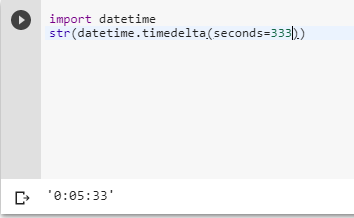2 views
in Python

I have a function that returns information in seconds, but I need to store that information in hours:minutes:seconds.

Is there an easy way to convert the seconds to this format in Python?

by (106k points)

There are many ways to convert seconds into hours, minutes and seconds some the important methods I am mentioning here:-

The first and very short way of converting seconds into hours, minutes and seconds are to use  datetime.timedelta function. Below is the code example that illustrates the use of this function.

>>> import datetime

>>> str(datetime.timedelta(seconds=333))Another important method you can use is by using the divmod() function, the divmod() function only does a single division to produce both the quotient and the remainder, you can have the results very quickly with only two mathematical operations. Below is the code that illustrates the use of divmod() function. The below-mentioned code uses string formatting to convert the result into your desired output.

divmod():-

The divmod() function takes two (non-complex) numbers as arguments and returns a pair of numbers consisting of their quotient and remainder when using integer division. For the integer numbers, the result is the same as (a // b, a % b)gives. When dealing with floating-point numbers the result is (q, a % b), where q is usually math.floor(a / b) but maybe 1 less than that. In any case q * b + a % b is very close to a, if a % b is non-zero it has the same sign as b, and 0 <= abs(a % b) < abs(b).

m, s = divmod(s, 60)

h, m = divmod(m, 60)

print(f'{h:d}:{m:02d}:{s:02d}')